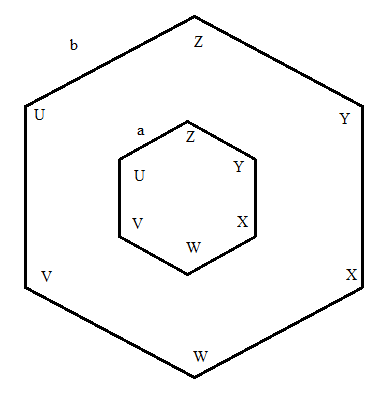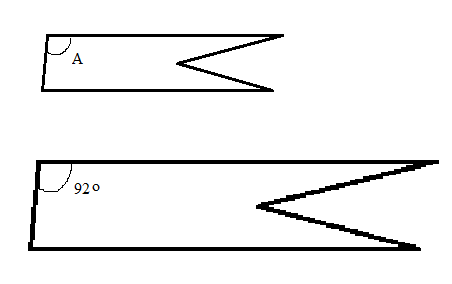# Similar Polygons: Practice Problems - Quiz & Worksheet

Instructions:

question 1 of 3

### In a pair of similar polygons, which is true?

Create Your Account To Take This Quiz

As a member, you'll also get unlimited access to over 84,000 lessons in math, English, science, history, and more. Plus, get practice tests, quizzes, and personalized coaching to help you succeed.

Try it risk-free for 30 days. Cancel anytime

### 1. The two hexagons shown are similar. Angles are labeled in capital letters. The perimeter of the larger hexagon is 20 feet, and the perimeter of the smaller hexagon is 10 feet. What is a in terms of b?### 2. The two polygons shown are similar. What is the measure of angle A?Create your account to access this entire worksheet
Quizzes, practice exams & worksheets
Certificate of Completion
Create an account to get started

Try this multiple-choice quiz at any time to discover how much you know about similar polygons. Problems you'll be covering address the characteristics of two similar polygons and comparing two hexagons of different sizes.

## Quiz & Worksheet Goals

These points will be addressed on the quiz:

• Measure of angles when comparing two similar polygons
• Similar polygons where the perimeter of the smaller shape is a given portion of the larger shape's perimeter
• True statements about similar polygons

## Skills Practiced

• Distinguishing differences - compare and contrast polygons that are similar in size
• Knowledge application - use your knowledge of similar polygons to complete practice problems
• Information recall - access the knowledge you've gained regarding characteristics of similar polygons

Consider the lesson named Similar Polygons: Practice Problems for more information on the following topics:

• Corresponding angles in similar polygons
• Simplifying diagrams
Final ExamGlencoe Geometry: Online Textbook Help
Status: Not Started
Chapter ExamGlencoe Geometry Chapter 6: Proportions and Similarity
Status: Not Started

Support